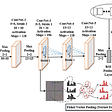# Review — Gaussian Error Linear Units (GELUs)

## GELU, Outperforms ReLU and ELU, in CV, NLP and Speech Tasks

Gaussian Error Linear Units (GELUs)
GELU, by University of California, and Toyota Technological Institute at Chicago
2016 arXiv, Over 600 Citations (Sik-Ho Tsang @ Medium)
Activation Unit, Image Classification, POS Tagging, Phone Recognition

• The GELU nonlinearity weights inputs by their value, rather than gates inputs by their sign as in ReLUs.
• Performance improvements are obtained across all considered computer vision, natural language processing, and speech tasks.

# Outline

1. Gaussian Error Linear Unit (GELU)
2. Experimental Results

# 1. Gaussian Error Linear Unit (GELU)

• ReLU deterministically multiplying the input by zero or one and Dropout stochastically multiplying by zero.

Specifically, the neuron input x can be multiplied by m~Bernoulli(Φ(x)), where Φ(x) = P(X≤x); X~N(0, 1) is the cumulative distribution function of the standard normal distribution.

This distribution is chosen since neuron inputs tend to follow a normal distribution, especially with Batch Normalization.

• Since the cumulative distribution function of a Gaussian is often computed with the error function, the Gaussian Error Linear Unit (GELU) is defined as:
• The above equation is approximated as:
• or:
• if greater feedforward speed is worth the cost of exactness.
• Different N(μ, σ) can be used as CDF, but in this paper, N(0, 1) is used.

# 2. Experimental Results

## 2.1. MNIST Classification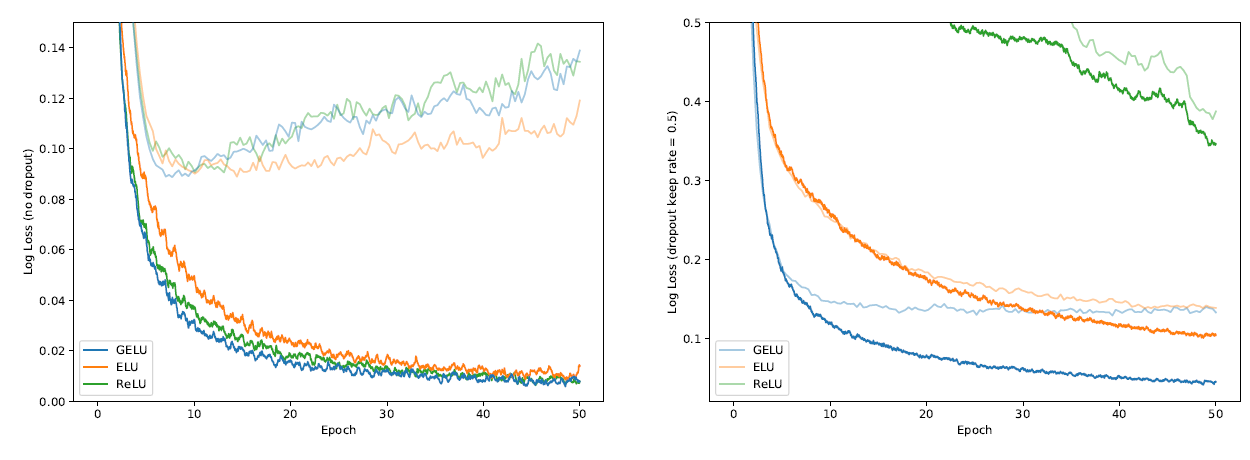MNIST Classification Results. Left are the loss curves without Dropout, and right are curves with a Dropout rate of 0.5.
• Fully connected networks with 8-layer, 128 neuron wide neural network are trained for 50 epochs with a batch size of 128.

GELU tends to have the lowest median training log loss with and without Dropout.

## 2.2. MNIST Autoencoder

• A deep autoencoder is trained on MNIST using self-supervised setting.
• The network is with layers of width 1000, 500, 250, 30, 250, 500, 1000, in order.

GELU accommodates different learning rates and significantly outperforms the other nonlinearities.

## 2.3. TIMIT Frame Classification

• Phone recognition with the TIMIT dataset which has recordings of 680 speakers in a noiseless environment.
• The system is a 5-layer, 2048-neuron wide classifier with 39 output phone labels and a Dropout rate of 0.5.

After five runs per setting, median test error chosen at the lowest validation error is 29.3% for the GELU, 29.5% for the ReLU, and 29.6% for the ELU.

## 2.4. CIFAR-10/100 Classification

• A shallower convolutional neural network of 9-layer is trained to test CIFAR-10. Each curve is a median of three runs.

On CIFAR-10, ultimately, the GELU obtains a median error rate of 7.89%, the ReLU obtains 8.16%, and the ELU obtains 8.41%.

• On CIFAR-100, WRN with 40 layers and a widening factor of 4 is trained.
• Over three runs we obtain the median convergence curves are shown above.

On CIFAR-100, the GELU achieves a median error of 20.74%, the ReLU obtains 21.77%, and the ELU obtains 22.98%.

## Reference

[2016 arXiv] [GELU]
Gaussian Error Linear Units (GELUs)

--

--

--

## More from Sik-Ho Tsang

PhD, Researcher. I share what I've learnt and done. :) My LinkedIn: https://www.linkedin.com/in/sh-tsang/, My Paper Reading List: https://bit.ly/33TDhxG

Love podcasts or audiobooks? Learn on the go with our new app.

## Introduction to Natural Language Processing## Naive Bayes For Text analysis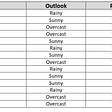## Part 2 — How I Tackled My First Kaggle Challenge Using Deep Learning## Dog Breed Prediction using Convolutional Neural Network## Neural ODE for Reinforcement Learning and Nonlinear Optimal Control: Cartpole Problem Revisited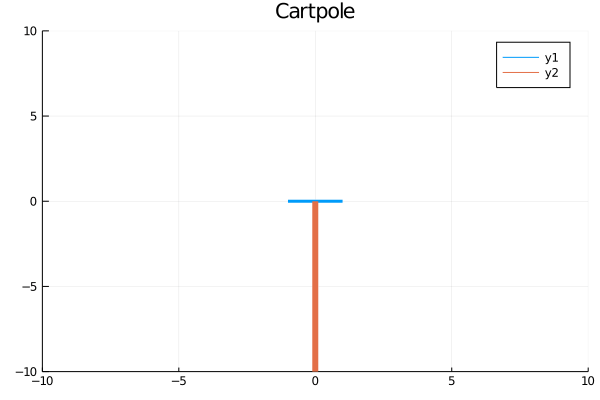## Review — Split-Brain Autoencoders: Unsupervised Learning by Cross-Channel Prediction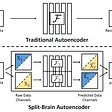## Reading: Kuanar JCCSP’19 — Object Detection Approach for Video Coding (Fast HEVC Prediction)